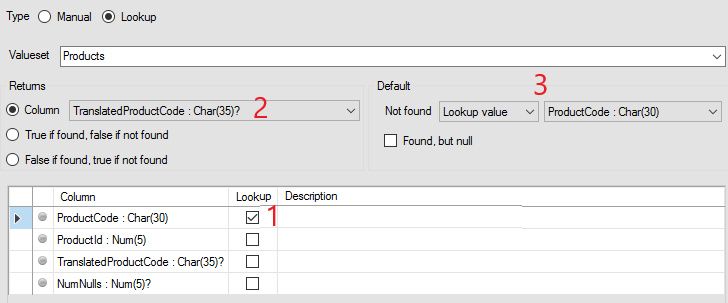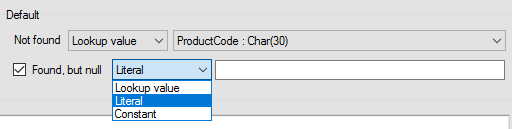Start a new topic

## Defining a Lookup rule in Studio

 This is a detailed topic in our support portal in the Using migFx series and assumes that you have some prior knowledge or experience using migFx. If you want to know more about hopp tech and migFx, our comprehensive solution for complex data migration, please visit our web site hopp.tech.

All Rules and Bag Methods in Studio comes in 2 flavours: Manual and Lookup. When defining a Manual Rule, you must specify the parameters, flags and flag parameters of the rule manually as well as provide a textual specification so it is clear what the Rule is supposed to be doing. Later you or someone else must implement the Rule manually (in c#).

However, when defining a Lookup Rule, you specify how you want a lookup in a Valueset to take place. migFx will then automatically generate the Rule implementation.

When defining a Lookup Rule, you specify the following:

• The Valueset the Rule is to lookup a row from
• Identify the columns to use for the lookup. These automatically become input parameters to the Rule
• What the Rule should return. You have these options
• Return the value of a Column in row that was found by the Lookup
• Return True if a row was found and False if no row was found
• Return False if a row was found and True if no row was found
• If the rule returns the value of a column, you can decide a default value, if no row was found
• A Lookup value. This allows you to choose one of the Lookup columns. The rule will return the input value for this Lookup column if no row found
• A Literal
• A Constant
• Null
• If the Column to return is of type Boolean, the options for the default value are instead
• True
• False
• A Lookup value
• A constant
• Finally, if the rule return the value of a Column, and this column is of a nullable data type, you can similarly choose what to return if a row was found, but the value of the Return column was null

All Lookup Rule are automatically created with 2 flags:

2. Found

Let's have a look at some use cases to make this clearer.

A simple Mapping Rule

The simplest use case is a matter of looking up a row using one or more lookup columns and return the value of another column on the row. If no row is found, then return Null.1 The Lookup is based on the Valueset Products 2 The Rule looks up up a value in the ProductCode column. migFx will generate an input parameter for the Rule and use the value for this parameter for the lookup 3 If a row is found by the lookup, the rule will raise flag 1 and return the value for the column ProductId 4 If no row is found, the rule will raise the flag 2 and return null

A Translation Rule

In another use case, the aim is to lookup the row using one or more lookup columns (that become parameters to the rule) and return the value of another column in order to translate the value of one of the input parameters. If no row is found, simply return the value of the input parameter itself. In this way, translation only takes place if a row is found.1 The Rule looks up up a value in the ProductCode column. migFx will generate an input parameter for the Rule and use the value for this parameter for the lookup 2 If a row is found by the lookup, the rule will raise flag 1 and return the value for the column TranslateProductCode 3 If no row is found, the rule will raise the flag 2 and return the value of the input parameter ProductCode

When the default Lookup value is selected, the corresponding drop down will list only the available columns:

• Marked as Lookup
• With a data type corresponding to the selected Returns column

Found, but null

In the above example, if a row is found, but the value of TranslateProductCode in the row is null, the rule will return this null value anyway. If that is not the desired behavior, another default return value can be defined for this situation by checking the Found, but null checkbox:Returning a Boolean column the old way

Early versions of Studio had fewer options for default return values. If a rule is meant to return true if a row is found and false if no row is found, then is was common to use this technique:1 A Boolean column is added to the Valueset, the column Found in this case. It is ensured that all rows in the Valueset contains the value True in this column 2 If a row is found by the lookup, the rule will raise flag 1 and return the value for the column Found (which of course will be True) 3 If no row is found, the rule will raise the flag 2 and return the default value False. Note that the options in the default value drop down reflects that the data type of the return column is Boolean